# ISEE Lower Level Math : How to find the missing part of a list

## Example Questions

1 3 Next →

### Example Question #81 : Data Analysis

What is the value of x in the following set?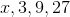Explanation:

Given that each subsequent number is 3 times the previous number, the value of x is 1, because 1 times 3 is 3. Therefore, 1 is the correct answer.

### Example Question #21 : How To Find The Missing Part Of A List

What is the value of x in the set below?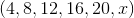Explanation:

The pattern in the set is that each number increases by 4. Therefore, since 4 plus 20 equals 24, x is 24.

### Example Question #22 : Sets

What is the missing value in the following set: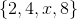Explanation:

The set is made up of only even numbers that are below.  The only missing even number that is less thanfrom this set would be.

### Example Question #23 : Sets

The follow set is all the outcomes of rolling a die. What is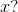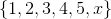Explanation:

Since a die has six sides, all of the possible outcomes arethrough.

Sinceis the only value in that range missing from the set,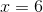.

### Example Question #24 : Sets

If the set below includes all possible whole number factors of, what is missing?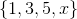Explanation:

A factor is a number that is multiplied by another to get another value.

To getyou may multiply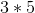and also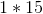.

With that in mind, that answer must be.

### Example Question #25 : Sets

Find the missing part to the set: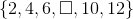Explanation:

When finding the missing part of a set, we must first look at the relationship each number has within the set.  So, looking at the setwe will look at the numbers in the set.  We can see the first three numbers are 2, 4, and 6.  How are these numbers related?  We can see that from one number to the next, we are adding 2 (if we add 2 to 2, we get 4; if we add 2 to 4 we get 6).  Let's look at the last two numbers, 10 and 12.  Does that same relationship work?  Yes, we are again adding 2 to 10 and we get 12.

So, to find the missing number in the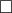, we will simply add 2 to the number before it.  So,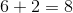Therefore, the missing number in the set is.

1 3 Next →

### All ISEE Lower Level Math Resources